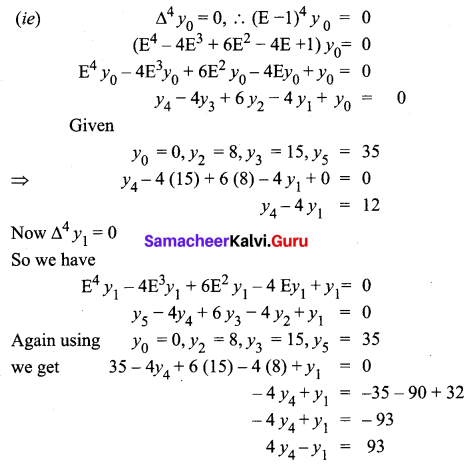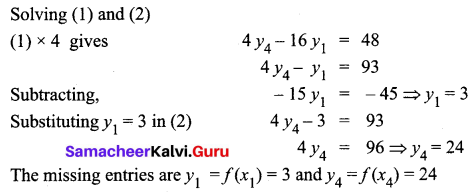# Samacheer Kalvi 12th Business Maths Solutions Chapter 5 Numerical Methods Ex 5.1

Students can download 12th Business Maths Chapter 5 Numerical Methods Ex 5.1 Questions and Answers, Samacheer Kalvi 12th Business Maths Book Solutions Guide Pdf helps you to revise the complete Tamilnadu State Board New Syllabus and score more marks in your examinations.

## Tamilnadu Samacheer Kalvi 12th Business Maths Solutions Chapter 5 Numerical Methods Ex 5.1

Question 1.
Evaluate (log ax)
Solution: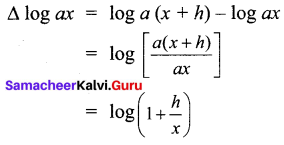Question 2.
If y = x3 – x2 + x – 1 calculate the values of y for x = 0, 1, 2, 3, 4, 5 and form the forward differences table.
Solution:
Given y = x3 – x2 + x – 1
When
x = 0, y = 0 – 0 + 0 – 1 = -1
x = 1, y = 1 – 1 + 1 – 1 = 0
x = 2, y = 8 – 4 + 2 – 1 = 5
x = 3, y = 27 – 9 + 3 – 1 = 20
x = 4, y = 64 – 16 + 4 – 1 = 51
x = 5, y = 125 – 25 + 5 – 1 = 104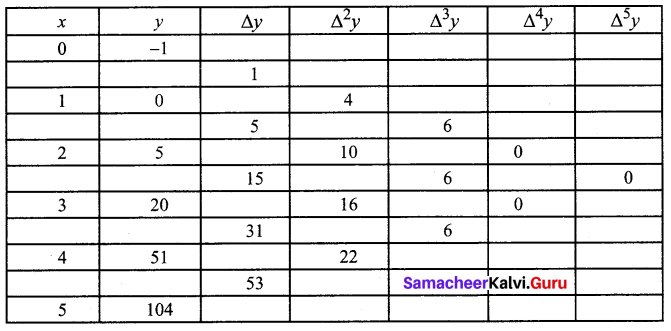Question 3.
If h = 1 then prove that (E-1 ∆) x3 = 3x2 – 3x + 1.
Solution:
h = 1 To prove (E-1 ∆) x3 = 3×2 – 3x + 1
L.H.S = (E-1 ∆) x3 = E-1 (∆x3)
= E-1[(x + h)3 – x3]
= E-1( x + h)3 – E-1(x3)
= (x – h + h)3 – (x – h)3
= x3 – (x – h)3
But given h = 1
So(E-1 ∆) x3 = x3 – (x – 1)3
= x3 – [x3 – 3x2 + 3x – 1]
= 3x2 – 3x + 1
= RHSQuestion 4.
If f(x) = x2 + 3x then show that ∆f(x) = 2x + 4
Solution:
f(x) = x2 + 3 x
∆f(x) = f(x + h) – f(x)
= (x + h)2 + 3(x + h) – x2 – 3x
= x2 + 2xh + h2 + 3x + 3h – x2 – 3x
= 2xh + 3h + h2
Put h = 1, ∆f(x) = 2x + 4

Question 5.
Evaluate $$\Delta\left[\frac{1}{(x+1)(x+2)}\right]$$ by taking ‘1’ as the interval of differencing.
Solution: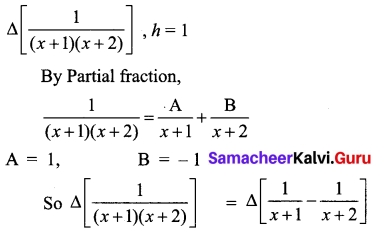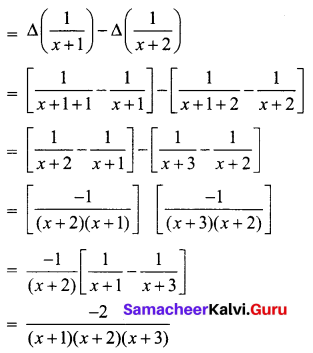Question 6.
Find the missing entry in the following table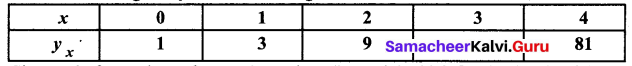Solution:
Since only four values of y are given, the polynomial which fits the data is of degree three. Hence fourth differences are zeros.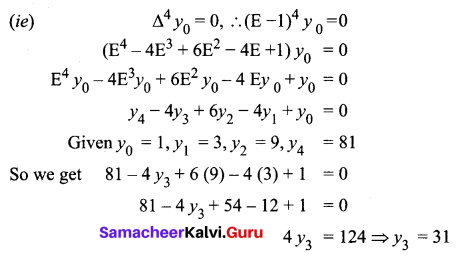Question 7.
Following are the population of a district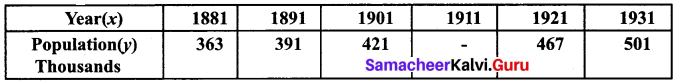Find the population of the year 1911?
Solution:
Since five values are given, the polynomial which fits the data is of degree four.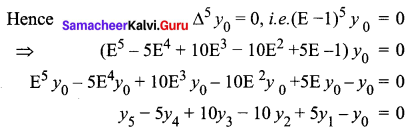From the given table
y0 = 363, y1 = 391, y2 = 421, y4 = 467 and y5 = 501
501 – 5(467) + 10y3 – 10(421) + 5(391) – 363 = 0
501 – 2335 + 10y3 – 4210 + 1955 – 363 = 0
-501 + 2335 + 4210 – 1955 + 363 = 10y3
10y3 = 4452
y3 = 445.2
Hence the population of the year 1911 is 445 thousandQuestion 8.
Find the missing entries from the following.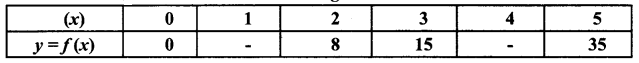Solution:
Since only four values of f(x) are given, the polynomial which fits the data is of degree three. Hence fourth differences are zeros.
Solution: